Previous: Font Shape  Up: Text Style  Next: Anchor

## Text Transformation Matrix

In addition to scaling, rotating and shearing a text area, you can also directly modify the transformation matrix using Edit->Text->Transformation Matrix. The transformation matrix for a text area is applied relative to the top left corner of the canvas. The transformation matrix for a text-path is applied relative to the point along the underlying path where each character should be positioned.

Once a text area has been combined with a path to form a text-path, the text can only be transformed by editing the transformation matrix. For example, Figure 9.19 shows a text-path in edit mode (so that you can see the underlying path) with different values of the transformation matrix. In Figure 9.19(a), the transformation matrix is set to the identity matrix, shown in Figure 9.19(b). In Figure 9.19(c), the transformation matrix is set as shown in Figure 9.19(d), (the vertical translation has been set to 10, which is in terms of the storage unit). The text is no longer flush against the path. In Figure 9.19(e), the transformation matrix has been set as shown in Figure 9.19(f), (the horizontal shear element has been set to -1).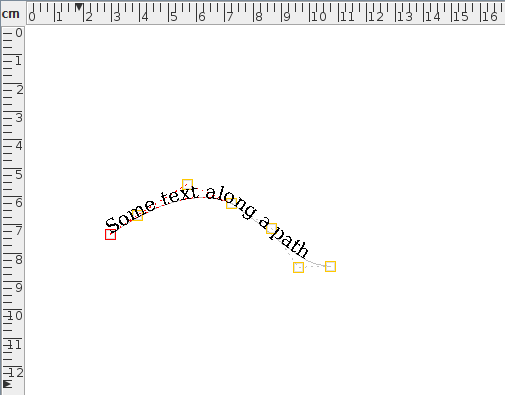(a)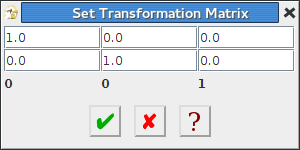(b)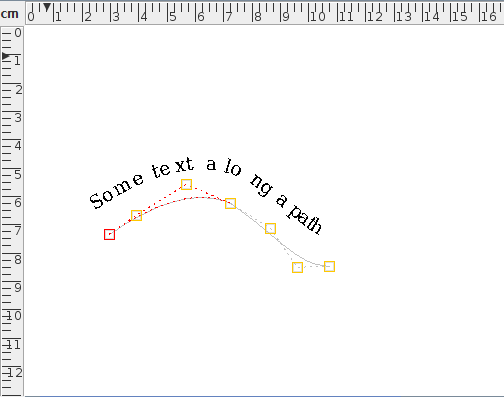(c)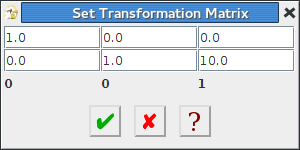(d)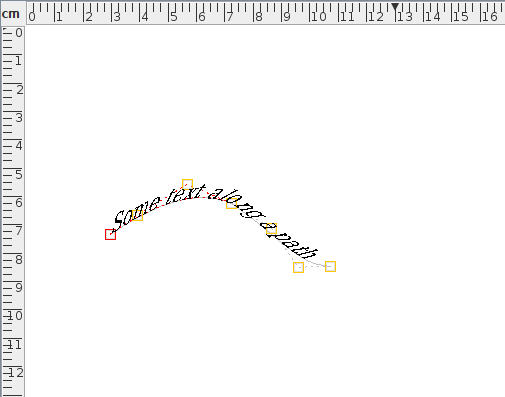(e)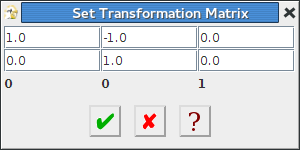(f)
Figure 9.19: Text-path transformation matrix: (a) the text-path with transformation matrix shown in (b); (c) the text-path with transformation matrix shown in (d); (e) the text-path with transformation matrix shown in (f).

The transformation matrix can be reset via the Transform->Reset Matrix menu item. This will reset the scale and shear factors (but not the translation) for text areas, and will reset all elements for text-paths.

Previous: Font Shape  Up: Text Style  Next: Anchor

© 2014 Dickimaw Books. "Dickimaw", "Dickimaw Books" and the Dickimaw parrot logo are trademarks. The Dickimaw parrot was painted by Magdalene Pritchett.